# Max-P Regionalization¶

Authors: Sergio Rey, Xin Feng, James Gaboardi

The max-p problem involves the clustering of a set of geographic areas into the maximum number of homogeneous regions such that the value of a spatially extensive regional attribute is above a predefined threshold value. The spatially extensive attribute can be specified to ensure that each region contains sufficient population size, or a minimum number of enumeration units. The number of regions $$p$$ is endogenous to the problem and is useful for regionalization problems where the analyst does not require a fixed number of regions a-priori.

Originally formulated as a mixed-integer problem in Duque, Anselin, Rey (2012), max-p is an NP-hard problem and exact solutions are only feasible for small problem sizes. As such, a number of heuristic solution approaches have been suggested. PySAL implements the heuristic approach described in Wei, Rey, and Knaap (2020).

:

%load_ext watermark
%watermark

Last updated: 2021-07-17T12:19:17.560741-04:00

Python implementation: CPython
Python version       : 3.9.6
IPython version      : 7.19.0

Compiler    : Clang 11.1.0
OS          : Darwin
Release     : 20.5.0
Machine     : x86_64
Processor   : i386
CPU cores   : 8
Architecture: 64bit


:

from spopt.region import MaxPHeuristic as MaxP
import matplotlib.pyplot as plt

import geopandas
import libpysal
import matplotlib
import numpy
import spopt
import warnings

plt.rcParams["figure.figsize"] = [12, 8]
warnings.filterwarnings("ignore")

RANDOM_SEED = 123456

%config InlineBackend.figure_format = "retina"
%watermark -w
%watermark -iv

Watermark: 2.1.0

geopandas : 0.9.0
libpysal  : 4.3.0
spopt     : 0.1.2
numpy     : 1.19.5
json      : 2.0.9
matplotlib: 3.3.3



## Mexican State Regional Income Clustering¶

To illustrate maxp we utilize data on regional incomes for Mexican states over the period 1940-2000, originally used in Rey and Sastré-Gutiérrez (2010).

We can first explore the data by plotting the per capital gross regional domestic product (in constant USD 2000 dollars) for each year in the sample, using a quintile classification. Here we will define a function for creating subplots useful in visual comparisons, which also can solve Max-P instances and will be used again later).

:

def subplotter(gdf, incrs, W, threshold=5, top_n=2, seed=RANDOM_SEED):
"""Helper plotting function, also solves MaxP instances if desired."""
rows, cols = incrs.shape
f, axs = plt.subplots(rows, cols, figsize=(9, 14))
for i in range(rows):
for j in range(cols):
year, _ax = incrs[i, j], axs[i, j]
if not year:
# plot country geographies
_attr = "Mexico"
gdf.plot(ax=_ax, ec="grey", fc="white")
else:
_attr = "PCGDP%s" % year
if not W:
# plot country geographies by attributes
plt_kws = dict(scheme="Quantiles", cmap="GnBu", ec="grey")
plt_kws.update(dict(legend_kwds={"fmt": "{:.0f}"}))
gdf.plot(column=_attr, ax=_ax, legend=True, **plt_kws)
else:
# solve a MaxP instance and plot regions
numpy.random.seed(seed)
maxp_args = gdf, W, _attr, "count", threshold
model = MaxP(*maxp_args, top_n=top_n)
model.solve()
label = year+"labels_"
gdf[label] = model.labels_
gdf.plot(column=label, ax=_ax, cmap="tab20")
_ax.set_title(_attr)
_ax.set_axis_off()
_ax.set_aspect("equal")
plt.tight_layout()

:

pth = libpysal.examples.get_path("mexicojoin.shp")

:

POLY_ID AREA CODE NAME PERIMETER ACRES HECTARES PCGDP1940 PCGDP1950 PCGDP1960 ... GR9000 LPCGDP40 LPCGDP50 LPCGDP60 LPCGDP70 LPCGDP80 LPCGDP90 LPCGDP00 TEST geometry
0 1 7.252751e+10 MX02 Baja California Norte 2040312.385 1.792187e+07 7252751.376 22361.0 20977.0 17865.0 ... 0.05 4.35 4.32 4.25 4.40 4.47 4.43 4.48 1.0 MULTIPOLYGON (((-113.13972 29.01778, -113.2405...
1 2 7.225988e+10 MX03 Baja California Sur 2912880.772 1.785573e+07 7225987.769 9573.0 16013.0 16707.0 ... 0.00 3.98 4.20 4.22 4.39 4.46 4.41 4.42 2.0 MULTIPOLYGON (((-111.20612 25.80278, -111.2302...
2 3 2.731957e+10 MX18 Nayarit 1034770.341 6.750785e+06 2731956.859 4836.0 7515.0 7621.0 ... -0.05 3.68 3.88 3.88 4.04 4.13 4.11 4.06 3.0 MULTIPOLYGON (((-106.62108 21.56531, -106.6475...
3 4 7.961008e+10 MX14 Jalisco 2324727.436 1.967200e+07 7961008.285 5309.0 8232.0 9953.0 ... 0.03 3.73 3.92 4.00 4.21 4.32 4.30 4.33 4.0 POLYGON ((-101.52490 21.85664, -101.58830 21.7...
4 5 5.467030e+09 MX01 Aguascalientes 313895.530 1.350927e+06 546702.985 10384.0 6234.0 8714.0 ... 0.13 4.02 3.79 3.94 4.21 4.32 4.32 4.44 5.0 POLYGON ((-101.84620 22.01176, -101.96530 21.8...

5 rows × 35 columns

:

mxgdp_years = [str(x) for x in range(1940, 2010, 10)]
mxgdp_years = numpy.array([None]+mxgdp_years)
mxgdp_years_grid = mxgdp_years.reshape(4,2)
mxgdp_years_grid

:

array([[None, '1940'],
['1950', '1960'],
['1970', '1980'],
['1990', '2000']], dtype=object)


### Regional incomes for Mexican states over the period 1940-2000¶

:

subplotter(mexico, mxgdp_years_grid, None)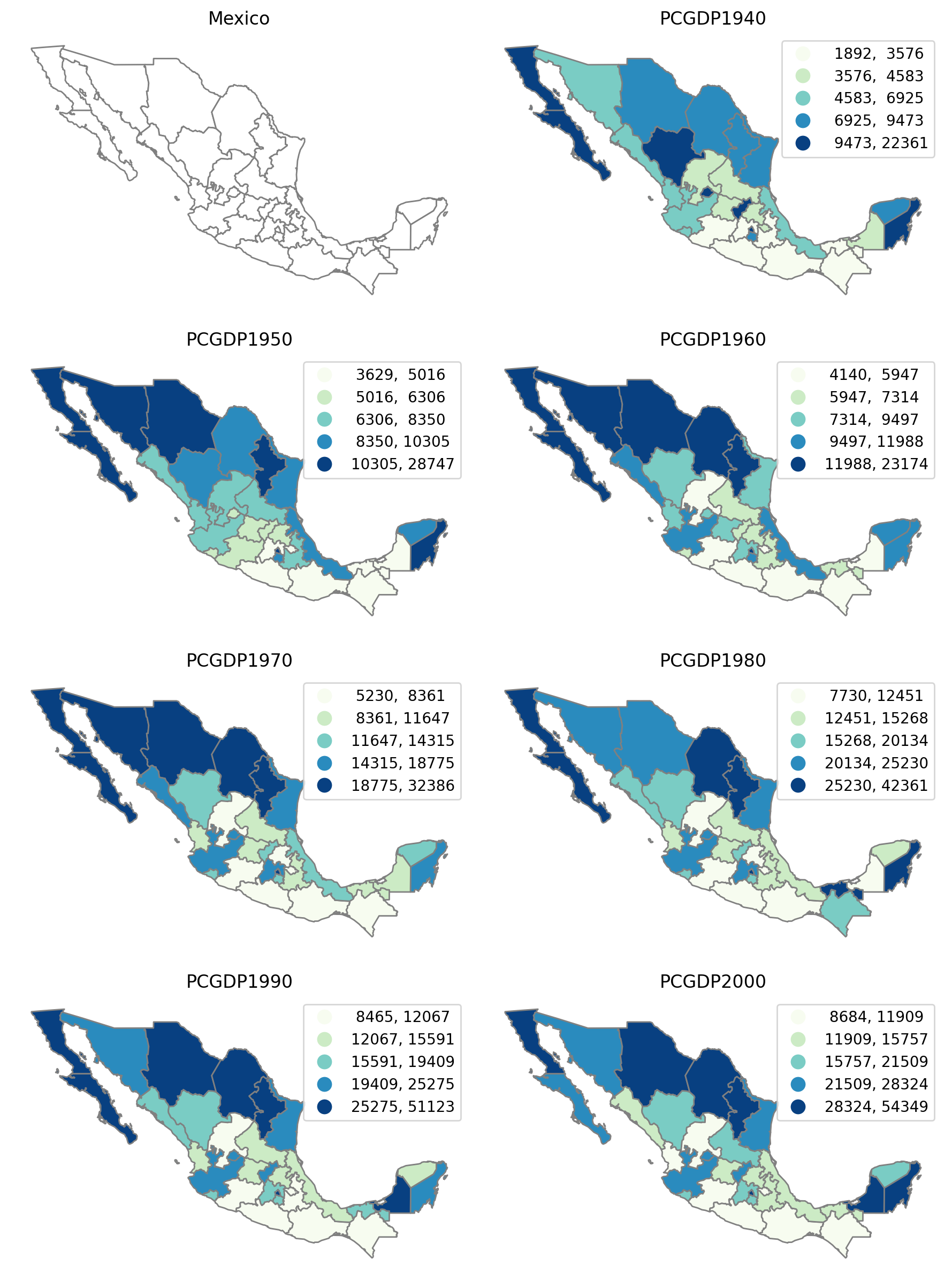In general terms, the north-south divide in incomes is present in each of the 7 decades. There is some variation in states moving across quintiles however, and this is true at both the bottom and top of the state income distribution.

To develop a holistic view of the Mexican space economy over this timespan, we can try to form a set of spatially connected regions that maximizes the internal socieconomic levels of the states belonging to each region.

## Pooled Regionalization¶

To develop our holistic view, we can treat the six cross-sections as a multidimensional array and seek to cluster 32 Mexican states into the maximum number of regions such that each region as at least 6 = 32 // 5 states and homogeneity in per capita gross regional product over 1940-2000 is maximized.

We first define the variables in the dataframe that will be used to measure regional homogeneity:

:

attrs_name = [f"PCGDP{year}" for year in mxgdp_years[1:]]
attrs_name

:

['PCGDP1940',
'PCGDP1950',
'PCGDP1960',
'PCGDP1970',
'PCGDP1980',
'PCGDP1990',
'PCGDP2000']


Next, we specify a number of parameters that will serve as input to the max-p model.

A spatial weights object expresses the spatial connectivity of the states:

:

w = libpysal.weights.Queen.from_dataframe(mexico)


The remaining arguments are the minimum number of states each region must have (threshold):

:

threshold = 6


and the number of the top candidate regions to consider when assigning enclaves (top_n):

:

top_n = 2


We create the attribute count which will serve as the threshold attribute which we add to the dataframe:

:

mexico["count"] = 1
threshold_name = "count"


The model can then be instantiated and solved:

:

numpy.random.seed(RANDOM_SEED)
model = MaxP(mexico, w, attrs_name, threshold_name, threshold, top_n)
model.solve()

:

mexico["maxp_new"] = model.labels_

:

mexico[["maxp_new", "AREA"]].groupby(by="maxp_new").count()

:

AREA
maxp_new
1 6
2 7
3 6
4 7
5 6
:

model.p

:

5

:

mexico.plot(column="maxp_new", cmap="tab20",  edgecolor="w");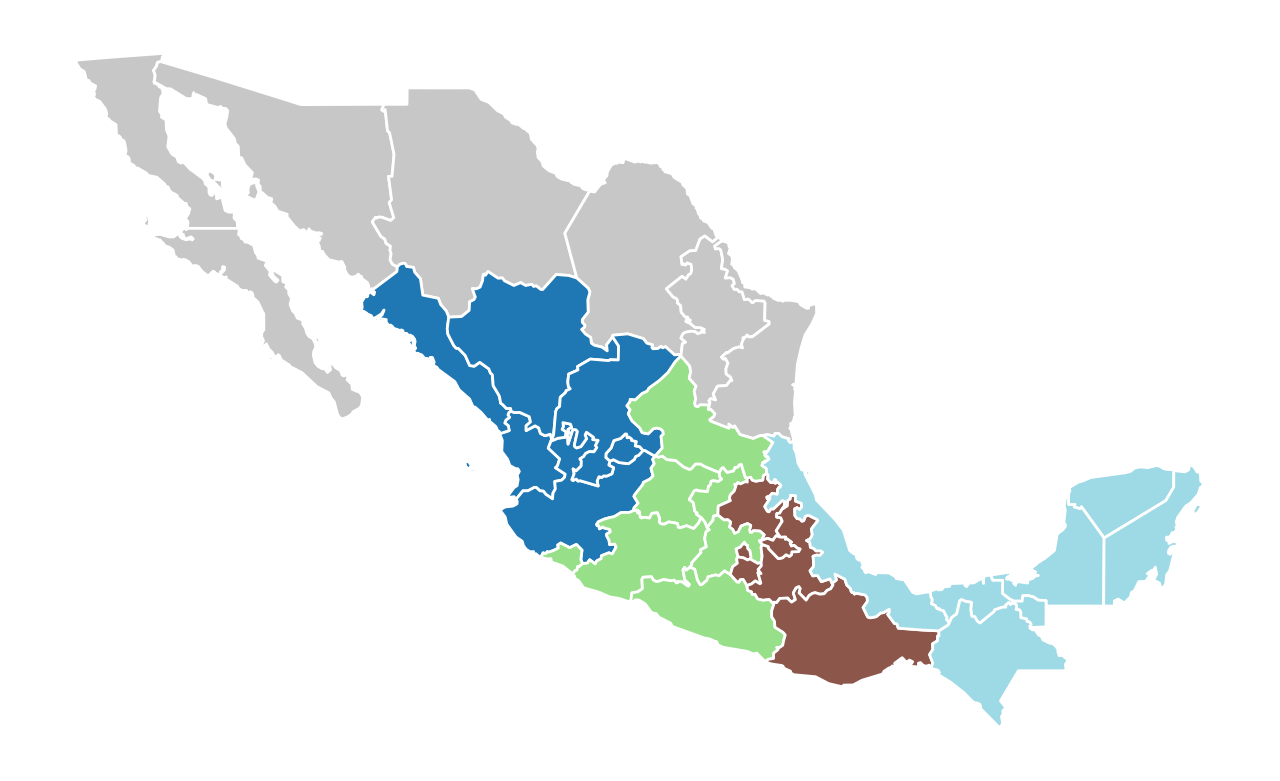The model solution results in five regions, three of which have six states, and two with seven states each. Each region is a spatially connected component, as required by the max-p problem.

## Change threshold to a minimum of 3 states per region¶

:

numpy.random.seed(RANDOM_SEED)
threshold = 3
model = MaxP(mexico, w, attrs_name, threshold_name, threshold, top_n)
model.solve()

:

mexico["maxp_3"] = model.labels_
mexico[["maxp_3","AREA"]].groupby(by="maxp_3").count()

:

AREA
maxp_3
1 3
2 3
3 4
4 3
5 3
6 3
7 4
8 3
9 3
10 3
:

model.p

:

10

:

mexico.plot(column="maxp_3", cmap="tab20", edgecolor="w");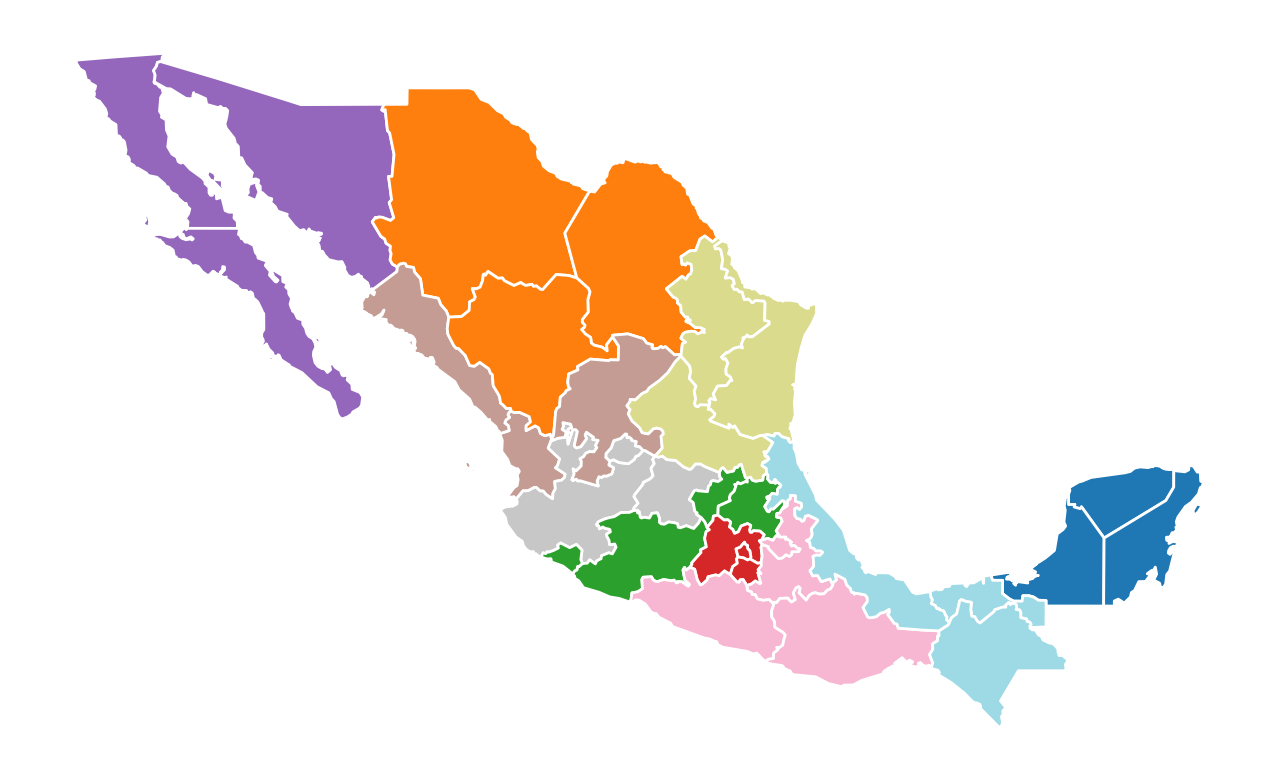## Year-by-Year Regionalization while Varying Parameters¶

5 states

:

region_args = mexico, mxgdp_years_grid, w

:

subplotter(*region_args, threshold=5, top_n=2)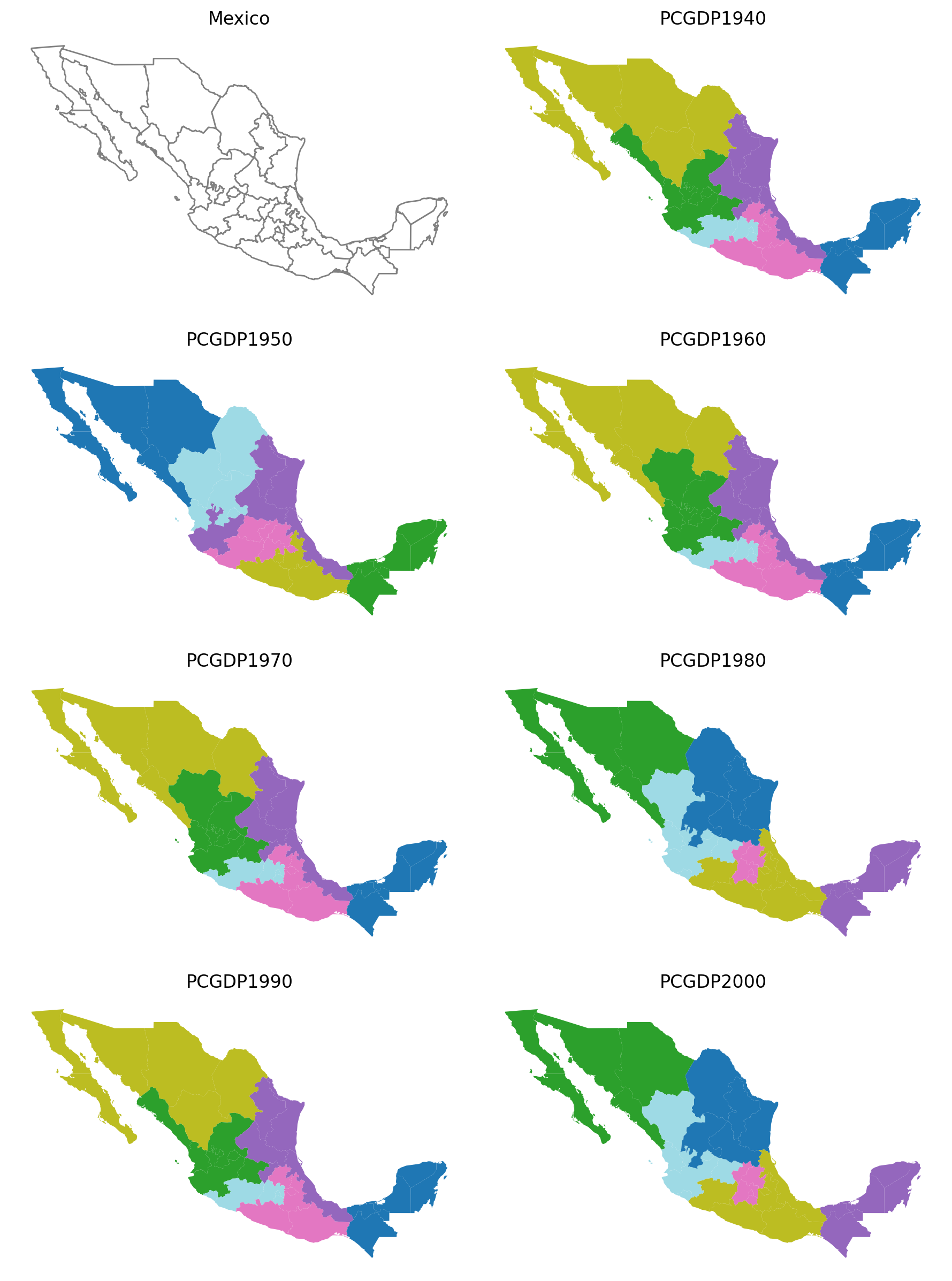10 states

:

subplotter(*region_args, threshold=10, top_n=2)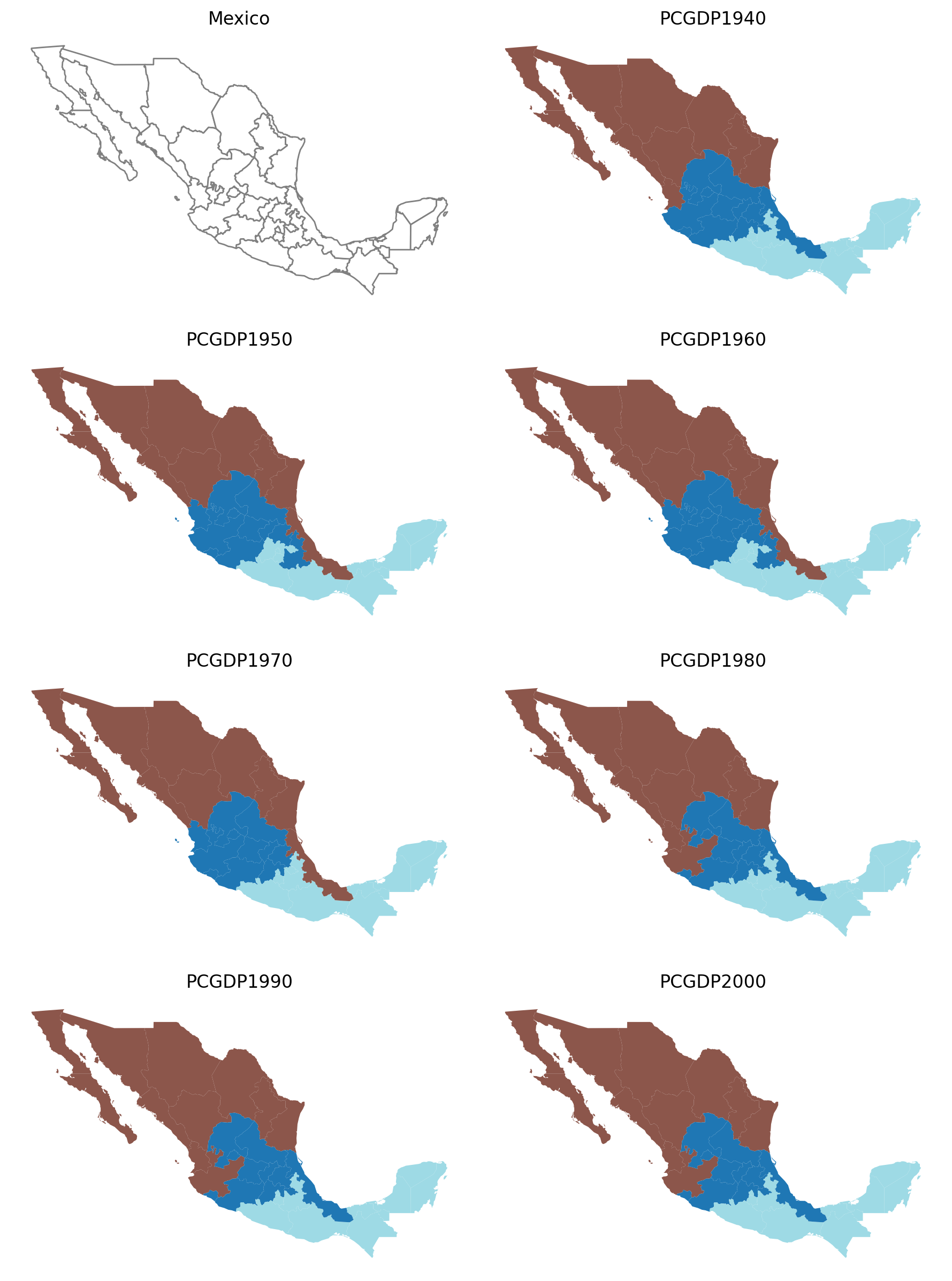15 states

:

subplotter(*region_args, threshold=15, top_n=2)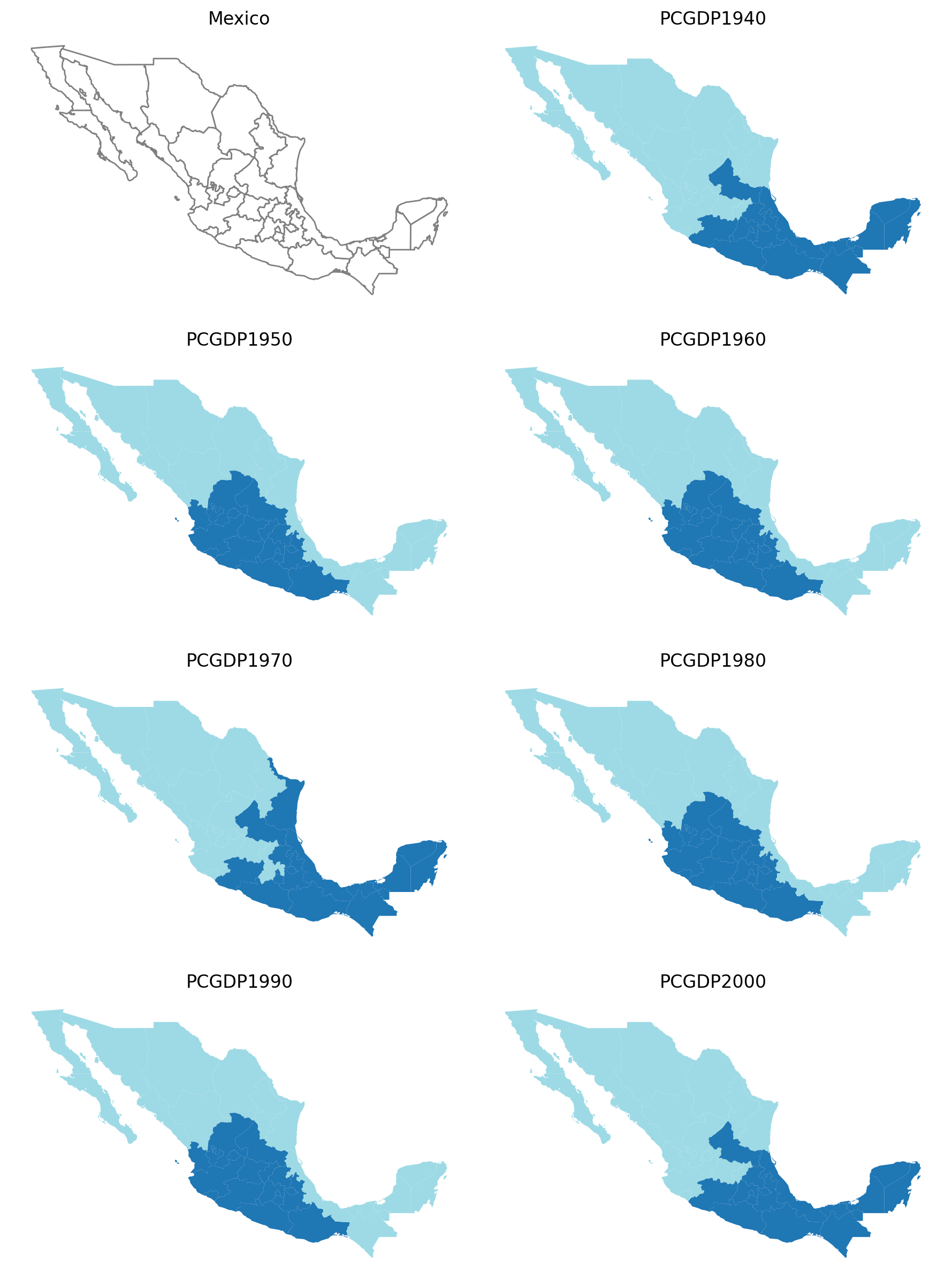1 state

:

subplotter(*region_args, threshold=5, top_n=1)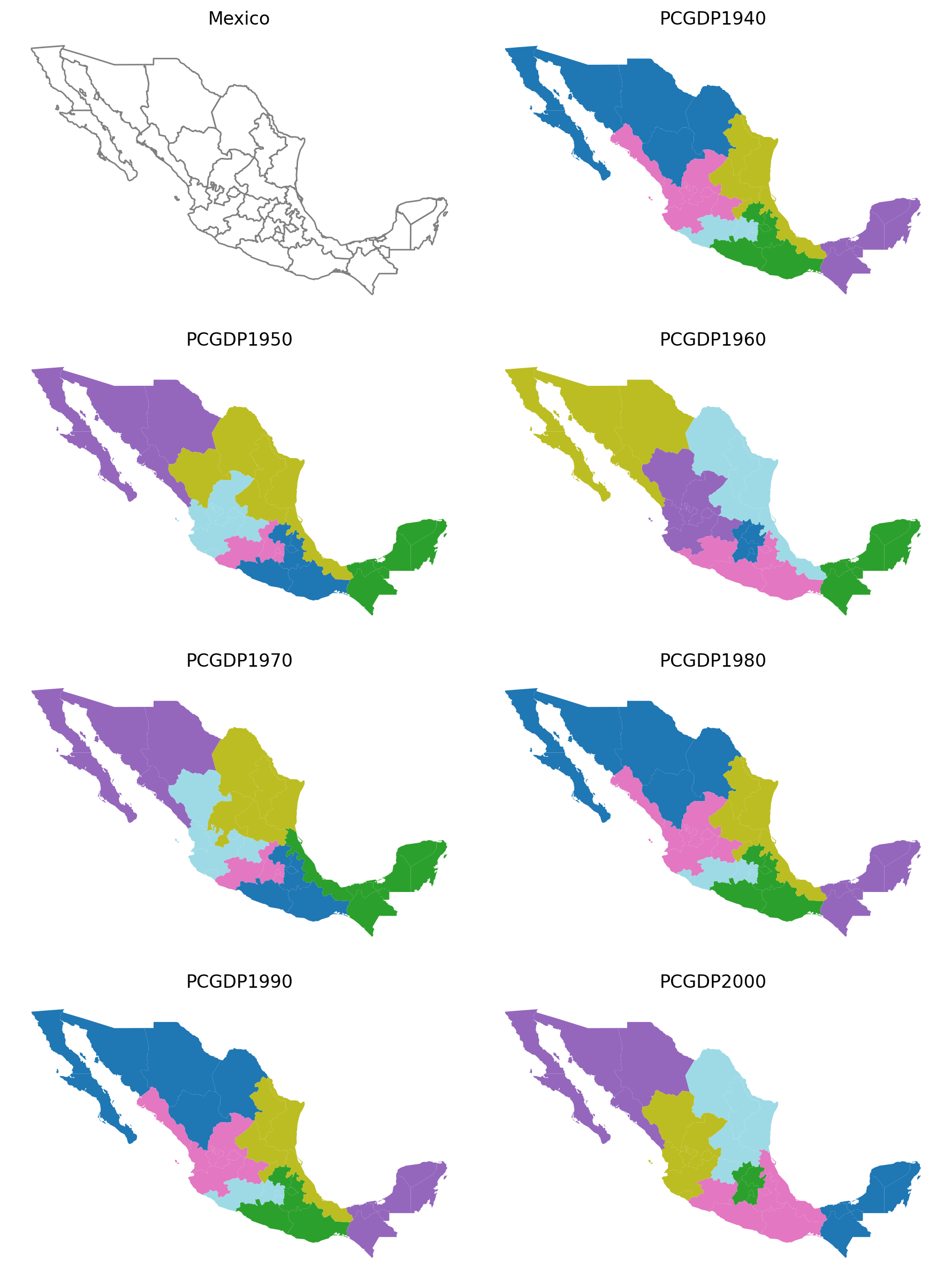3 states

:

subplotter(*region_args, threshold=5, top_n=3)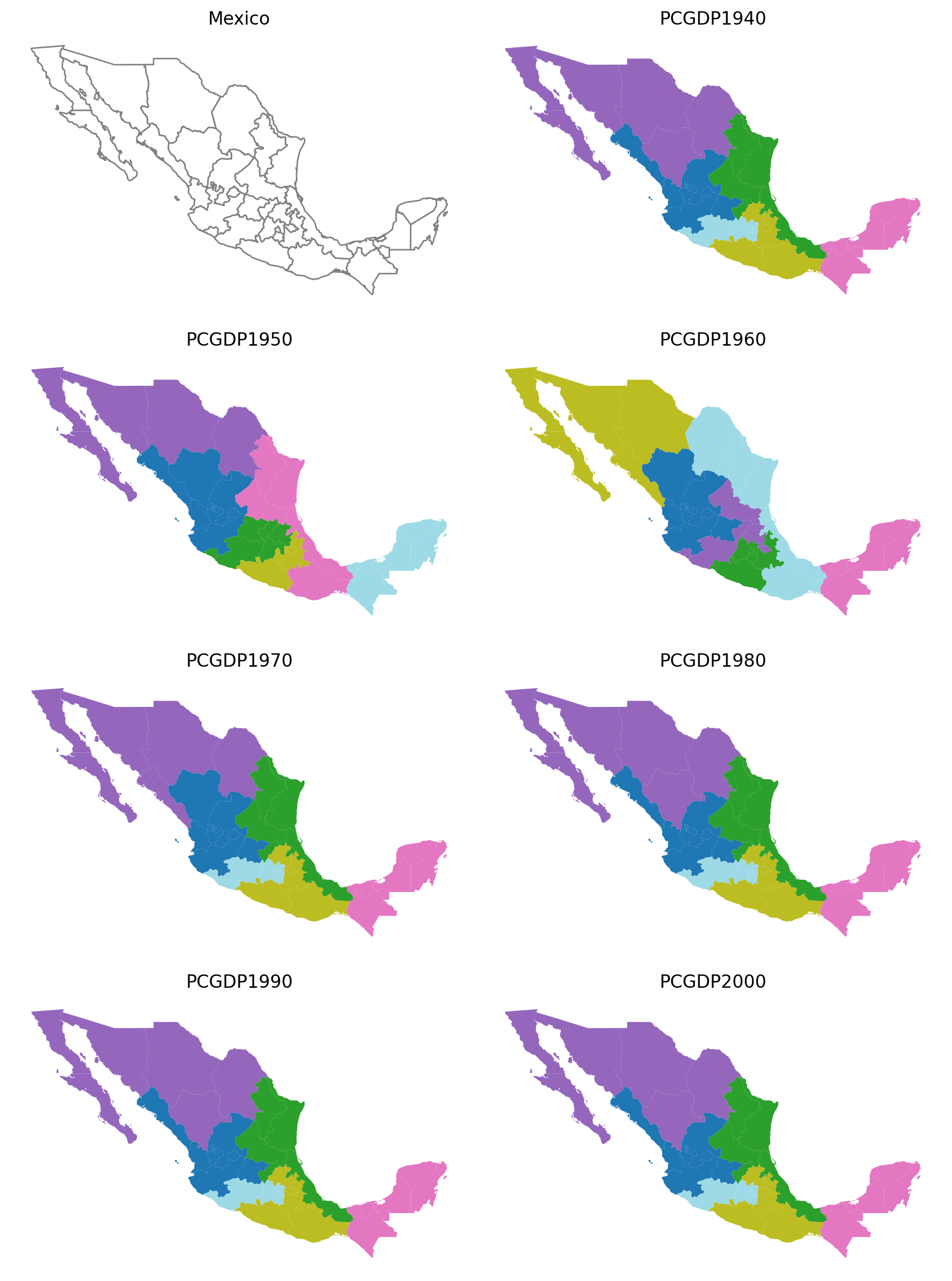5 states

:

subplotter(*region_args, threshold=5, top_n=5)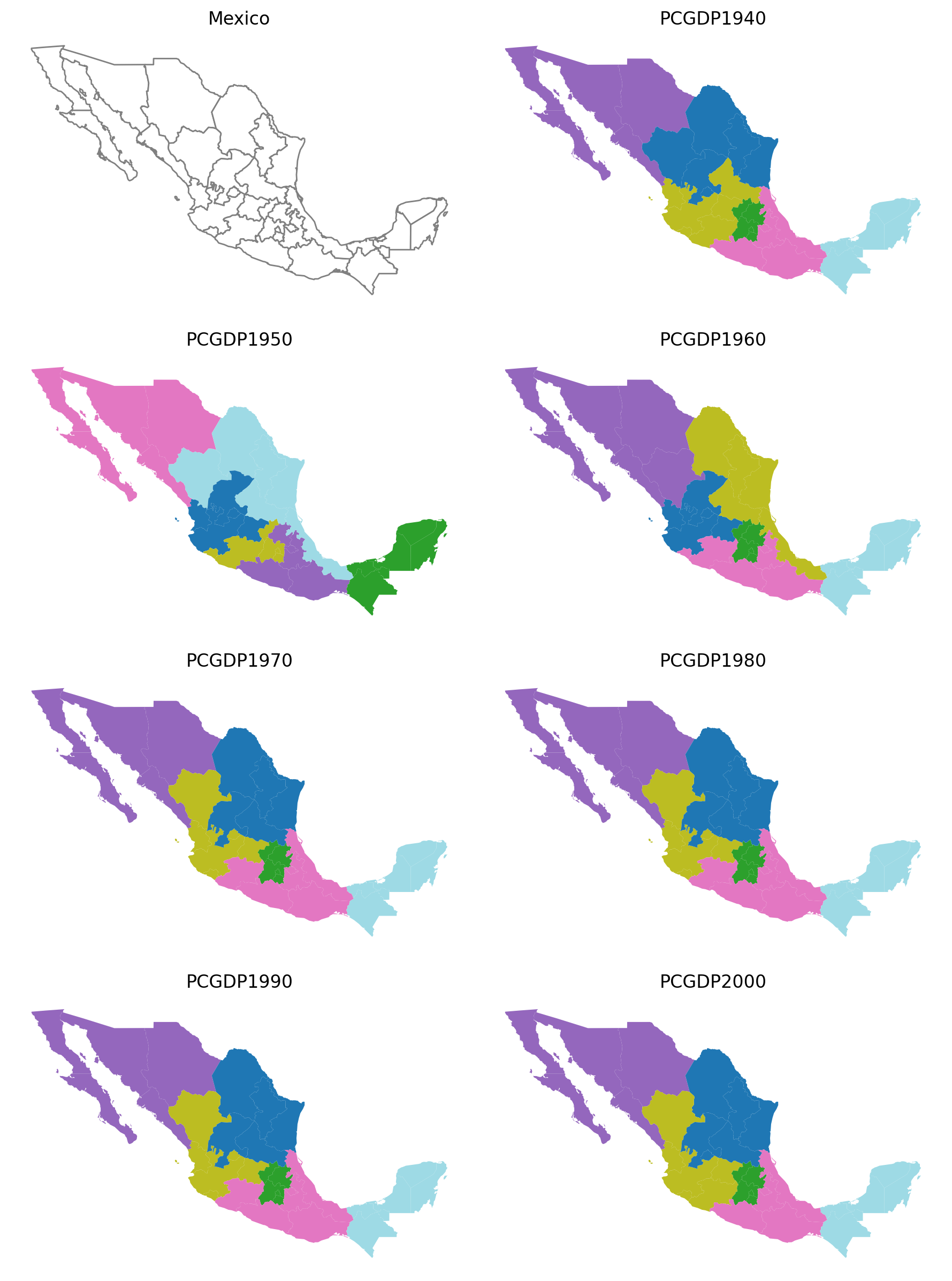As shown here, varying the threshold and top_n parameters both have a demonstrable effect regionalization outcomes.